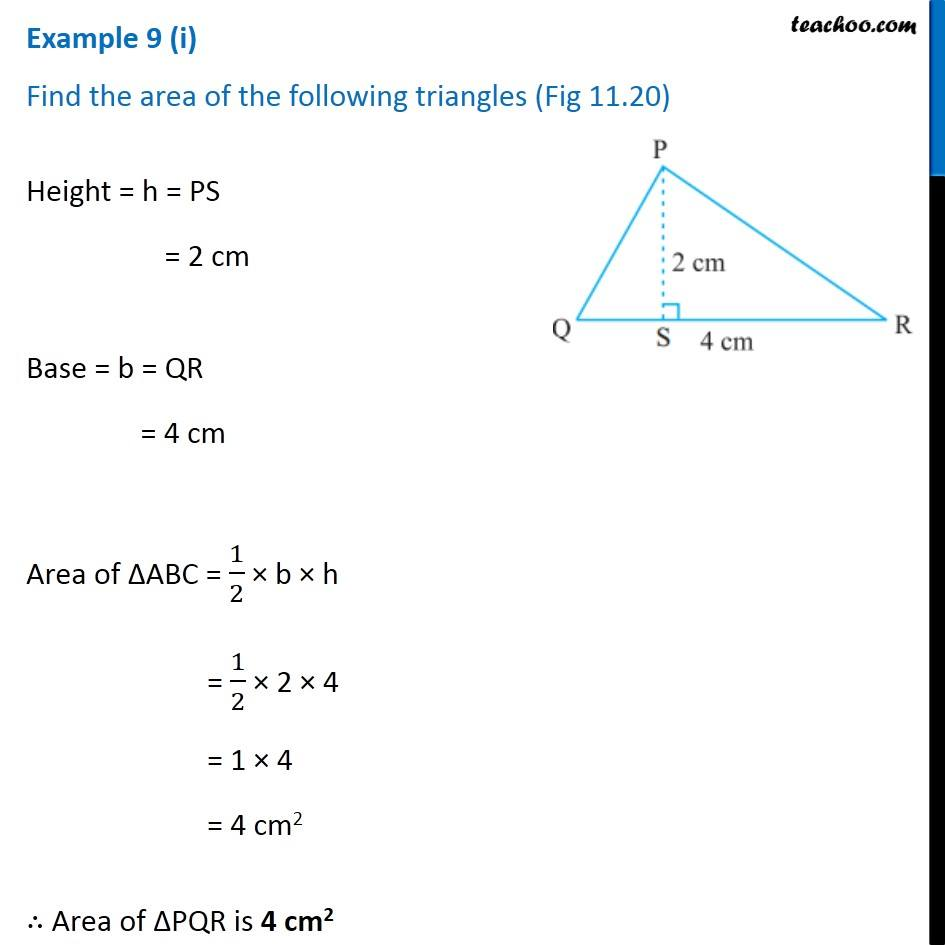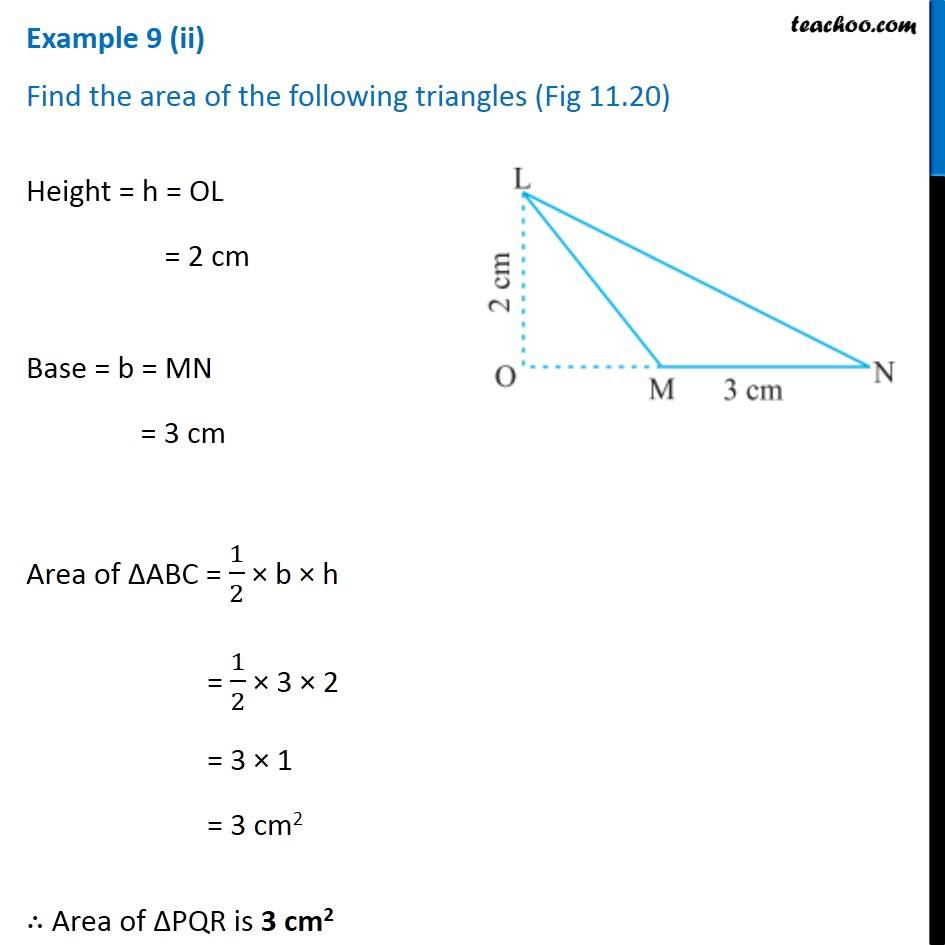Subscribe to our Youtube Channel - https://you.tube/teachoo

1. Chapter 11 Class 7 Perimeter and Area
2. Serial order wise
3. Examples

Transcript

Example 9 (i) Find the area of the following triangles (Fig 11.20) Height = h = PS = 2 cm Base = b = QR = 4 cm Area of ∆ABC = 1/2 × b × h = 1/2 × 2 × 4 = 1 × 4 = 4 cm2 ∴ Area of ∆PQR is 4 cm2 Example 9 (ii) Find the area of the following triangles (Fig 11.20) Height = h = OL = 2 cm Base = b = MN = 3 cm Area of ∆ABC = 1/2 × b × h = 1/2 × 3 × 2 = 3 × 1 = 3 cm2 ∴ Area of ∆PQR is 3 cm2

Examples